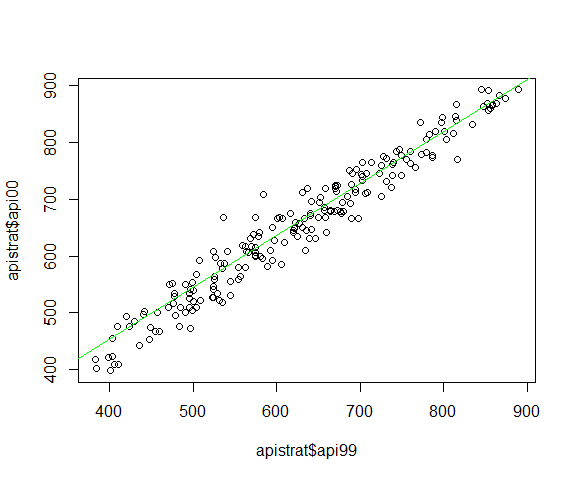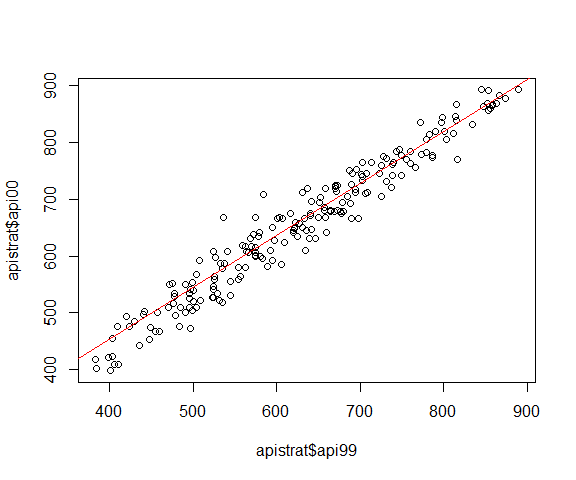# Introduction to robsurvey

## Introduction

The package robsurvey provides several functions to compute robust survey statistics. The package supports the computations of robust means, totals, and ratios. Available methods are Huber M-estimators, trimming, and winsorization.

All methods may be used with na.rm = TRUE in order to remove missing values before the computation starts.

Most of the methods provided in the package are described in (Hulliger 1995, Hulliger (1999), Hulliger et al. (2011), Templ et al. (2011)).

robsurvey complements the popular survey package.

Multivariate outlier detection and imputation methods can be found in the R-package modi.

## Overview

The main functions provided in robsurvey are:

• svymean_huber()
• svytotal_huber()
• svymean_trimmed()
• svytotal_trimmed()
• svymean_winsorized()
• svytotal_winsorized()
• weighted_line()
• weighted_median_line()
• weighted_median_ratio()

## Robust survey statistics

In the following example, we showcase a typical use of the package robsurvey. The data we use are from the package survey and describe the student performance in Californian schools. We will show different ways of how to compute a robust mean value for the Academic Performance Index (API) in 2000. The variable is denoted as api00. The following code chunk simply loads the data.

## load packages
library(robsurvey)
library(survey)

data(api)

The variables we are going to use are shown in the following table. sname denotes the name of the school and stype the type of school (E: elementary school, M: middle school, H: high school). As mentioned above, api00 denotes the Academic Performance Index of the school. pw is the weight of the observation and fpc is the population size that is used for the finite population correction. Note that the variable fpc is the same for all schools of the same type (E, M, H).

sname stype api00 pw fpc
Open Magnet: Center for Individual (Char E 840 44.21 4421
Belvedere Elementary E 516 44.21 4421
Altadena Elementary E 531 44.21 4421
Soto Street Elementary E 501 44.21 4421
Walnut Canyon Elementary E 720 44.21 4421
Atherwood Elementary E 805 44.21 4421

Based on the function svydesign from the survey package, we can now define a survey design for these data. With the argument strata we can stratify the dataset into the three types of schools.

# define survey design
dstrat <- svydesign(id = ~1, strata = ~stype, weights = ~pw,
data = apistrat, fpc = ~fpc)

Having defined the survey design, it is now very easy to compute robust survey statistics with the functions provided in robsurvey. In the following code chunk, we first compute the robust Horvitz-Thompson M-estimator for the mean API. In addition, we compute the trimmed and winsorized (robust) Horvitz-Thompson mean. Note how the estimates and the corresponding standard errors vary. The scale estimate used in the Huber-type robust M-estimator is the median absolute deviation (MAD), which is rescaled to be consistent at the normal distribution, i.e. it is multiplied by the constant 1.4826. The default tuning constant of the Huber-type Horvitz-Thompson M-estimator is k = 1.5. However, in this example we manually set it to k = 2.

# compute the robust Horvitz-Thompson M-estimator of the mean
svymean_huber(~api00, dstrat, k = 2)
#>          mean    SE
#> api00 662.907 8.926

# compute the robust trimmed Horvitz-Thompson mean
svymean_trimmed(~api00, dstrat, k = 2)
#>          mean     SE
#> api00 655.362 11.568

# compute the robust winsorized Horvitz-Thompson mean
svymean_winsorized(~api00, dstrat, k = 2)
#>          mean     SE
#> api00 640.599 11.568

It is also possible to use svymean_huber() (or analogously, svymean_trimmed() or svymean_winsorized()) in combination with svyby() from the survey package in order to compute the robust sample means for all three groups (or strata).

# Domain estimates
svyby(~api00, by = ~stype, design = dstrat, svymean_huber, k = 2)
#>   stype    api00       se
#> E     E 675.8750 11.72813
#> H     H 626.3659 13.59477
#> M     M 635.4545 15.05877

Instead of the mean, you may be interested in computing the robust total:

# compute the robust HTE for the total
svytotal_huber(~api00, dstrat, k = 2)
#>         total       SE
#> api00 4106045 55289.86

# compute the robust trimmed total
svytotal_trimmed(~api00, dstrat, k = 2)
#>         total       SE
#> api00 4059312 71652.89

# compute the robust winsorized total
svytotal_winsorized(~api00, dstrat, k = 2)
#>         total       SE
#> api00 3967868 71652.89

For simulations and as intermediate results, the above functions can also be used without the survey package. They then only deliver the bare estimate. We extract the weights from the survey design with weights(dstrat). Note that the estimate is identical to svymean_huber(~api00, dstrat, k = 2).

# bare-bone function to compute robust Horvitz-Thompson M-estimator of the mean
weighted_mean_huber(apistrat$api00, weights(dstrat), k = 2) #>  662.9068 ## Robust simple linear regression and ratio estimator A weighted version of the resistant line function of base R (line()) is provided as weighted_line(). # weighted resistant line out <- weighted_line(apistrat$api00 ~ apistrat$api99, w = weights(dstrat)) # what are coefficients? coef(out) #>  88.4729242 0.9133574 # plot data plot(apistrat$api00 ~ apistrat$api99) abline(out, col = "green")Two median-based simple regression estimators are provided (weighted_median_line()). One which takes the weighted median of the centered ratios as the estimator for the slope (type = "slopes") and one which replaces all sums in the least squares estimator formula for the slope by weighted medians (type = "products"). The weighted median of ratios estimators are $b_{1, slopes}=M\left(\frac{y-M(y, w)}{x-M(x, w)}, w\right)$ and $b_{0, slopes}=M(y-b_{1, slopes}\cdot x, w),$ where $$M(x, w)$$ is a weighted median of a vector $$x$$ weighted with $$w$$. The weighted median of products estimators are $b_{1, products}=\frac{M((y-M(y, w))(x-M(x, w)), w)}{M((x-M(x, w))^2, w)}$ and $b_{0, products}=M(y-b_{1, products}\cdot x, w).$ # weighted median line based on slopes out_s <- weighted_median_line(apistrat$api00 ~ apistrat$api99, w = weights(dstrat)) # weighted median line based on products out_p <- weighted_median_line(apistrat$api00 ~ apistrat$api99, w = weights(dstrat), type = "products") # what are coefficients? coef(out_s) #>  86.7647059 0.9159664 coef(out_p) #>  86.5587687 0.9163807 # plot data plot(apistrat$api00 ~ apistrat$api99) abline(out_s, col = "blue") abline(out_p, col = "red")A weighted regression through the origin based on medians is provided in weighted_median_ratio(). These functions may be useful for detecting bivariate outliers, as estimators on their own or as starting values for iterated M-estimators. ## Utility functions The robsurvey package provides several functions which may be used outside the package, in particular weighted_median() and weighted_quantile(). The full list of utility functions is as follows: • weighted_median() • weighted_quantile() • weighted_mad() • weighted_total() • weighted_mean() The weighted median calculates a median from the weighted empirical cumulative distribution function. Undefiniteness is solved by taking the mean of the low and the high median. Weighted quantiles use the lower quantile in case of undefiniteness. # compute weighted median for api00 weighted_median(apistrat$api00, weights(dstrat))
#>  668